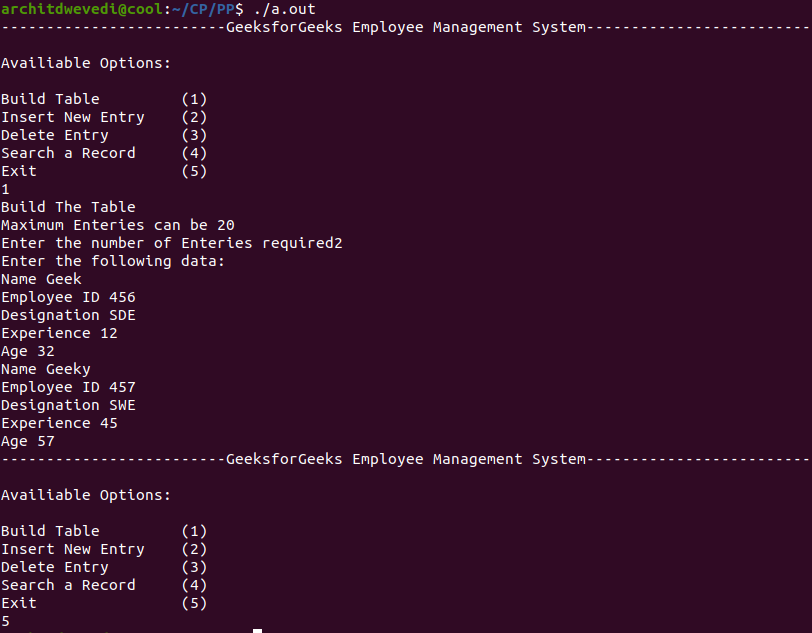# Program for Employee Management System

• Difficulty Level : Hard
• Last Updated : 06 Sep, 2021

A Employee’s Management System (EMS) is a software built to handle the primary housekeeping functions of a company. EMS help companies keep track of all the employees and their records. It is used to manage the company using computerized system.

Aim of Employee’s Management System:

Attention reader! All those who say programming isn't for kids, just haven't met the right mentors yet. Join the  Demo Class for First Step to Coding Coursespecifically designed for students of class 8 to 12.

The students will get to learn more about the world of programming in these free classes which will definitely help them in making a wise career choice in the future.

• Built The Employee Table.
• Insert New Entries.
• Delete The Entries.
• Search A Record.

Data of the Employee’s:

• Name
• Employee ID
• Designation
• Experience
• Age

Approach:

1. For storing the data of the employee, create a user define datatype which will store the information regarding Employee. Below is the declaration of the data type:
```struct employee {
string name;
long int code;
string designation;
int exp;
int age;
};
```
2. Building the Employee's table: For building the employee table the idea is to use the array of the above struct datatype which will use to store the information regarding employee. For storing information at index i the data is stored as:
```struct employee emp;
emp[i].name = "GeeksforGeeks"
emp[i].code = "12345"
emp[i].designation = "Organisation"
emp[i].exp = 10
emp[i].age = 10
```
3. Deleting in the record: Since we are using array to store the data, therefore to delete the data at any index shift all the data at that index by 1 and delete the last data of the array by decreasing the size of array by 1.
4. Searching in the record: For searching in the record based on any parameter, the idea is to traverse the data and if at any index the value parameters matches with the record stored, print all the information of that employee.

Below is the implementation of Employee Management system in C:

## C++

 `// C++ program for the above approach``#include `` ` `#define max 20``using` `namespace` `std;`` ` `// Structure of Employee``struct` `employee {``    ``string name;``    ``long` `int` `code;``    ``string designation;``    ``int` `exp``;``    ``int` `age;``};`` ` `int` `num;``void` `showMenu();`` ` `// Array of Employees to store the``// data in the form of the Structure``// of the Array``employee emp[max], tempemp[max],``    ``sortemp[max], sortemp1[max];`` ` `// Function to build the given datatype``void` `build()``{``    ``cout << ``"Build The Table\n"``;``    ``cout << ``"Maximum Entries can be "``         ``<< max << ``"\n"``;`` ` `    ``cout << ``"Enter the number of "``         ``<< ``"Entries required"``;``    ``cin >> num;`` ` `    ``if` `(num > 20) {``        ``cout << ``"Maximum number of "``             ``<< ``"Entries are 20\n"``;``        ``num = 20;``    ``}``    ``cout << ``"Enter the following data:\n"``;`` ` `    ``for` `(``int` `i = 0; i < num; i++) {``        ``cout << ``"Name "``;``        ``cin >> emp[i].name;`` ` `        ``cout << ``"Employee ID "``;``        ``cin >> emp[i].code;`` ` `        ``cout << ``"Designation "``;``        ``cin >> emp[i].designation;`` ` `        ``cout << ``"Experience "``;``        ``cin >> emp[i].``exp``;`` ` `        ``cout << ``"Age "``;``        ``cin >> emp[i].age;``    ``}`` ` `    ``showMenu();``}`` ` `// Function to insert the data into``// given data type``void` `insert()``{``    ``if` `(num < max) {``        ``int` `i = num;``        ``num++;`` ` `        ``cout << ``"Enter the information "``             ``<< ``"of the Employee\n"``;``        ``cout << ``"Name "``;``        ``cin >> emp[i].name;`` ` `        ``cout << ``"Employee ID "``;``        ``cin >> emp[i].code;`` ` `        ``cout << ``"Designation "``;``        ``cin >> emp[i].designation;`` ` `        ``cout << ``"Experience "``;``        ``cin >> emp[i].``exp``;`` ` `        ``cout << ``"Age "``;``        ``cin >> emp[i].age;``    ``}``    ``else` `{``        ``cout << ``"Employee Table Full\n"``;``    ``}`` ` `    ``showMenu();``}`` ` `// Function to delete record at index i``void` `deleteIndex(``int` `i)``{``    ``for` `(``int` `j = i; j < num - 1; j++) {``        ``emp[j].name = emp[j + 1].name;``        ``emp[j].code = emp[j + 1].code;``        ``emp[j].designation``            ``= emp[j + 1].designation;``        ``emp[j].``exp` `= emp[j + 1].``exp``;``        ``emp[j].age = emp[j + 1].age;``    ``}``    ``return``;``}`` ` `// Function to delete record``void` `deleteRecord()``{``    ``cout << ``"Enter the Employee ID "``         ``<< ``"to Delete Record"``;`` ` `    ``int` `code;`` ` `    ``cin >> code;``    ``for` `(``int` `i = 0; i < num; i++) {``        ``if` `(emp[i].code == code) {``            ``deleteIndex(i);``            ``num--;``            ``break``;``        ``}``    ``}``    ``showMenu();``}`` ` `void` `searchRecord()``{``    ``cout << ``"Enter the Employee"``         ``<< ``" ID to Search Record"``;`` ` `    ``int` `code;``    ``cin >> code;`` ` `    ``for` `(``int` `i = 0; i < num; i++) {`` ` `        ``// If the data is found``        ``if` `(emp[i].code == code) {``            ``cout << ``"Name "``                 ``<< emp[i].name << ``"\n"``;`` ` `            ``cout << ``"Employee ID "``                 ``<< emp[i].code << ``"\n"``;`` ` `            ``cout << ``"Designation "``                 ``<< emp[i].designation << ``"\n"``;`` ` `            ``cout << ``"Experience "``                 ``<< emp[i].``exp` `<< ``"\n"``;`` ` `            ``cout << ``"Age "``                 ``<< emp[i].age << ``"\n"``;``            ``break``;``        ``}``    ``}`` ` `    ``showMenu();``}`` ` `// Function to show menu``void` `showMenu()``{`` ` `    ``cout << ``"-------------------------"``         ``<< ``"GeeksforGeeks Employee"``         ``<< ``" Management System"``         ``<< ``"-------------------------\n\n"``;`` ` `    ``cout << ``"Available Options:\n\n"``;``    ``cout << ``"Build Table         (1)\n"``;``    ``cout << ``"Insert New Entry    (2)\n"``;``    ``cout << ``"Delete Entry        (3)\n"``;``    ``cout << ``"Search a Record     (4)\n"``;``    ``cout << ``"Exit                (5)\n"``;`` ` `    ``int` `option;`` ` `    ``// Input Options``    ``cin >> option;`` ` `    ``// Call function on the bases of the``    ``// above option``    ``if` `(option == 1) {``        ``build();``    ``}``    ``else` `if` `(option == 2) {``        ``insert();``    ``}``    ``else` `if` `(option == 3) {``        ``deleteRecord();``    ``}``    ``else` `if` `(option == 4) {``        ``searchRecord();``    ``}``    ``else` `if` `(option == 5) {``        ``return``;``    ``}``    ``else` `{``        ``cout << ``"Expected Options"``             ``<< ``" are 1/2/3/4/5"``;``        ``showMenu();``    ``}``}`` ` `// Driver Code``int` `main()``{`` ` `    ``showMenu();``    ``return` `0;``}`

Output:
Below is the output of the above program:My Personal Notes arrow_drop_up